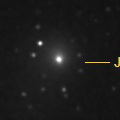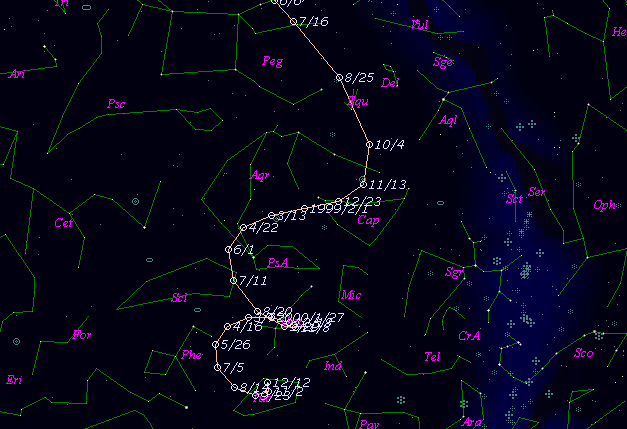# \$B%`!<%K%((B-\$B%G%e%W%\$WB@1(B

C/1997 J2 ( Meunier-Dupouy )

 English version Home page Updated on December 31, 2016###\$B%W%m%U%#!<%k(B

 \$BH/8+F|(B 1997\$BG/(B5\$B7n(B7\$BF|(B, 8\$BF|(B \$BH/8+8wEY(B 14.0\$BEy(B, 15.5\$BEy(B \$BH/8+ Michel Meunier (Arbonne la Foret), Philippe Dupouy, J. F. Lahitte (Observatoire de Dax)

###\$B###\$B50F;MWAG(B

```   The following improved orbital elements by Kenji Muraoka, are
from 1353 observations 1997 May 5 to 1999 Aug. 16, perturbations
by 8 Planets, Moon and 3 minor planets were taken into account.
The mean residual is +/- 0.69 arc seconds.

Epoch  =  1998 Mar.  8.0  TT        JDT = 2450880.5
T  =  1998 Mar. 10.44247       +/- 0.00044 (m.e.) TT
Peri. =  122.67413                +/- 0.00006
Node  =  148.84465                +/- 0.00004   (2000.0)
Incl. =   91.27334                +/- 0.00005
q  =    3.0511376              +/- 0.0000022 AU
e  =    1.0006137              +/- 0.0000058
1/a  =   -0.0002012              +/- 0.0000019 1/AU
orig. 1/a  =   +0.000040
fut.  1/a  =   -0.000002
```

###\$B@1?^(B###\$B8wEYJQ2=(B

```        m1 = 3.7 + 5 log\$B&\$(B + 10.0 log r  [   ,300]  (              \$B!A(B1999\$BG/(B 1\$B7n(B 4\$BF|(B)
m1 = 5.5 + 5 log\$B&\$(B + 10.0 log r  [300,   ]  (1999\$BG/(B 1\$B7n(B 4\$BF|!A(B              )
```##### \$B50F;MWAG\$OB<2,7r<#;a\$N7W;;\$K\$h\$k\$b\$N\$G\$9!#(B \$B@1?^\$O(B StellaNavigator Ver.2.0 for Windows (\$B%"%9%H%m%"!<%D(B \$BJTCx(B / \$B%"%9%-!<=PHG6I4)(B) \$B\$G:n@.\$7\$?\$b\$N\$G\$9!#(B \$B8wEY%0%i%U\$O(BComet for Windows\$B\$G:n@.\$7\$?\$b\$N\$G\$9!#(B